### Home > CC3 > Chapter 9 > Lesson 9.2.3 > Problem9-89

9-89.
1. Determine the positive value that makes each equation true. If the answer is not a whole number, write it as a square root, and then approximate it as a decimal rounded to the nearest tenth. Homework Help ✎

1. If x2 = 36, x = ?

2. If x2 = 65 , x = ?

3. If x2 = 84, x = ?

4. If x2 = 13, x = ?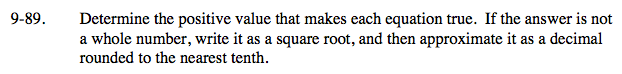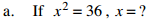What number multiplied by itself is 36?

${\it x} = \sqrt{36}$

x = 6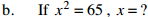Is 65 the square of any whole number?

$\sqrt{65} \text{ is approximately equal to 8.1.}$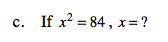Is 84 the square of any whole number?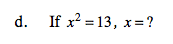Is 13 the square of any whole number?

x ≈ 3.6